# SOLIDWORKS Flow Simulation 2018 Introduces Free Surface Simulation (Part 1: Theory)Article by Siavash Khajehhasani created/updated September 28, 2017

SOLIDWORKS Flow Simulation 2018 allows you to model the flow of two immiscible fluids with a free surface. Liquids are considered as immiscible if they are completely insoluble in each other. A free surface is an interface between immiscible fluids, for example, a liquid and a gas (any pair of fluids belonging to gases, or liquids are allowed excluding gas-gas contact).

However, any phase transitions (including humidity, condensation, cavitation), rotation problems, surface tension and boundary layer on an interface between immiscible fluids are not allowed in this version.

Free surfaces are modeled with the Volume of Fluid (VOF) technique by solving a single set of momentum equations and tracking the volume fraction of each of the fluids throughout the domain.

The VOF technique is based on the concept of a fluid volume fraction αq (q = 0…Nq-1), which must have a value between 0 and 1. In a two-phase system, for example, in mesh cells (control volumes) of liquid α0 = 0 and α1 = 1, while in cells of gas α0 = 1 and α1 = 0. The location of a free surface is where αq changes from 0 to 1. In each control volume, the volume fractions of all phases sum to unity: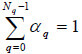(1) where Nq is the number of immiscible fluids (component phases).

Flow Simulation allows to model a two-phase system with Nq = 2 only. Pairs of liquid-gas or liquid-liquid are available.

The density in each cell is either purely representative of one of the phases or a mixture of the phases, depending upon the volume fraction values:(2) The absence of immiscible fluids mixing means that there is no convective transfer of mass, momentum, energy and other parameters through the free surface. Also there is no diffusion transfer of the mixture components. However there are diffusion fluxes for momentum, energy, and turbulent parameters. It is assumed that velocities, shear stresses, static pressures, temperatures and heat fluxes are equal at the interface between immiscible fluids.

The terms grad (ρ) are assumed to be negligible within each fluid. For a gas phase changes in density ρq are mainly related to temperature variations, not pressure:(3) It is assumed that a gas phase flow is low-compressible, that is Mach number M < 0.3.

At the free surface the ratio between immiscible fluids densities (or viscosities) may be large, e.g., for water to air the ratio between densities is about 103.

The volume fraction equation is written as follows:(4) Then as a consequence of the volume fraction equations, the conservation law for mass has the following form: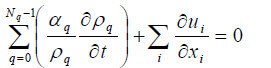(5) The momentum equation can be written as: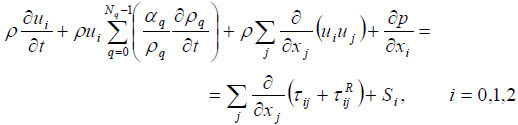(6) The energy equation can be written as: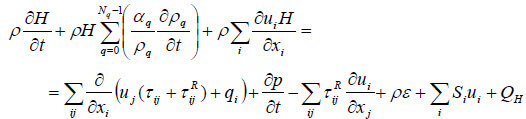(7) Each immiscible fluid is one substance, not a mixture.

The state equation of immiscible fluids has the following form: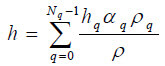(8) The other fluid properties (viscosity, heat conductivity and heat capacity) are defined in similar way: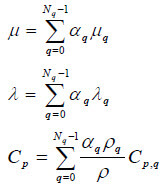(9) The free surface position can be restored at any time for visualization. The restored free surface position is not used in the numerical algorithm.

Reference: Technical Reference Document for SOLIDWORKS Flow Simulation 2018

### Posts related to 'SOLIDWORKS Flow Simulation 2018 Introduces Free Surface Simulation (Part 1: Theory)'

#### Find Related Content by TAG:### Siavash Khajehhasani

Siavash is a SOLIDWORKS Elite Applications Engineer and is based in our Oakville, Ontario office.